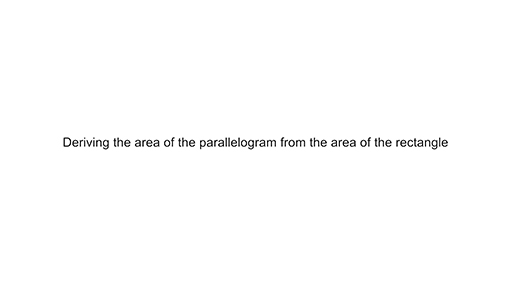Teaching mathematics

Start this free course now. Just create an account and sign in. Enrol and complete the course for a free statement of participation or digital badge if available.

Free course

# Using the area formula for a rectangle to derive other area formulas

The area formulas for parallelograms and triangles can be easily derived from the area formula of a rectangle (see Video 1).

Watch this video to see how the area formula of a rectangle can be used to derive the area of a parallelogram and then a triangle.

Download this video clip.Video player: Video 1 Deriving the area of a parallelogram and the area of a triangleSkip transcript: Video 1 Deriving the area of a parallelogram and the area of a triangle

#### Transcript: Video 1 Deriving the area of a parallelogram and the area of a triangle

INSTRUCTOR:
Step one, draw a rectangle. The area is a times b, which is length times width.
Step two, draw a line from one corner to the opposite side to make a triangle. Step three, cut out the triangle.
Step four, move the triangle to the other side to make a parallelogram. Area equals a times b, which is base times height of the parallelogram.
Step one, draw a parallelogram. Area equals base times height.
Step two, draw one of its diagonals. Step three, cuts along the diagonal.
Check the resulting two triangles are the same by placing one over the other. The area of one triangle is 1/2 the base times the height.
End transcript: Video 1 Deriving the area of a parallelogram and the area of a triangle
Video 1 Deriving the area of a parallelogram and the area of a triangle
Interactive feature not available in single page view (see it in standard view).

Since the parallelogram has been made by moving parts of the original rectangle without losing anything, the area of the parallelogram must be the same as the area of the rectangle.

It is worth bearing in mind that if the area formulas are promoted too quickly, before learners have conceptualised area as being a measure of the inside of a shape, then area measurement becomes a calculation without reference to the geometric situation.

TM_1